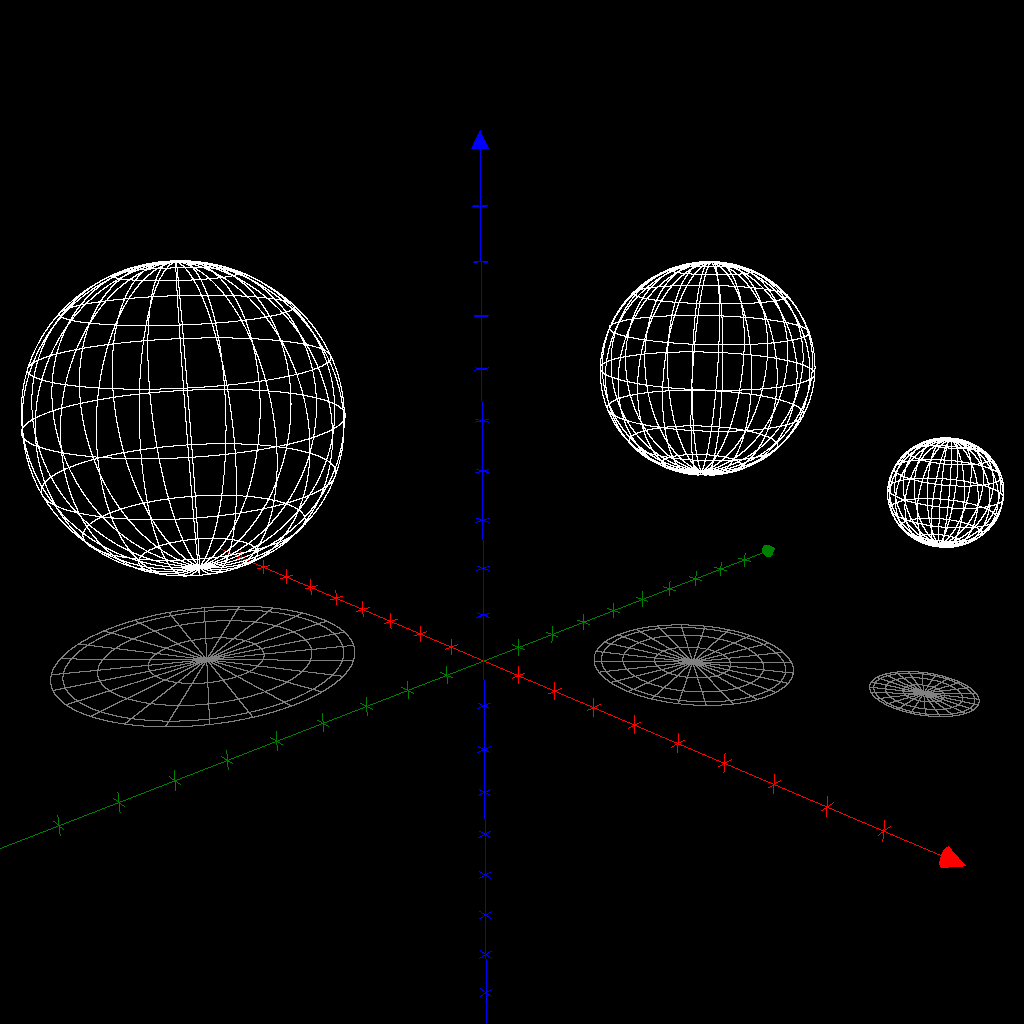# #AtoZChallenge2015: OrthogonalThe word orthogonal conjures up memories of wonderful maths classes, many years ago, as well as more obscure readings, much later, of absconse topological subjects. Geometry was one of the great pleasures of my youth: yes, we are all different!

I quote from: Barile, Margherita. “Orthogonal.” From MathWorld–A Wolfram Web Resource, created by Eric W. Weissteinhttp://mathworld.wolfram.com/Orthogonal.html

“In elementary geometry, orthogonal is the same as perpendicular. Two lines or curves are orthogonal if they are perpendicular at their point of intersection. Two vectorsandof the real planeor the real spaceare orthogonal iff their dot product. This condition has been exploited to define orthogonality in the more abstract context of the-dimensional real space.

More generally, two elementsandof an inner product spaceare called orthogonal if the inner product ofandis 0. Two subspacesandofare called orthogonal if every element ofis orthogonal to every element of. The same definitions can be applied to any symmetric or differential k-form and to any Hermitian form.”

For those of my readers so inclined, Wikipedia has an interesting article on matrices!

“In linear algebra, an orthogonal matrix is a square matrix with real entries whose columns and rows are orthogonal unit vectors (i.e., orthonormal vectors), i.e.$Q^\mathrm{T} Q = Q Q^\mathrm{T} = I,$

where I is the identity matrix.

This leads to the equivalent characterization: a matrix Q is orthogonal if its transpose is equal to its inverse:$Q^\mathrm{T}=Q^{-1}, \,$

An orthogonal matrix Q is necessarily invertible (with inverse Q−1 = QT), unitary (Q−1 = Q*) and therefore normal (Q*Q = QQ*) in the reals. The determinant of any orthogonal matrix is either +1 or −1. As a linear transformation, an orthogonal matrix preserves the dot product of vectors, and therefore acts as an isometry of Euclidean space, such as a rotation or reflection. In other words, it is a unitary transformation.

The set of n × n orthogonal matrices forms a group O(n), known as the orthogonal group. The subgroup SO(n) consisting of orthogonal matrices with determinant +1 is called the special orthogonal group, and each of its elements is a special orthogonal matrix. As a linear transformation, every special orthogonal matrix acts as a rotation.

The complex analogue of an orthogonal matrix is a unitary matrix.”

Image: orthogonal projection at http://english.rejbrand.se/algosim/visualisation.asp?id=orthogonal_projection

## One thought on “#AtoZChallenge2015: Orthogonal”

1.evelyneholingue

Sorry! I must have missed that one. Maybe it was meant to happen since geometry has never been my cup of tea! See you for letter S!

Like

This site uses Akismet to reduce spam. Learn how your comment data is processed.